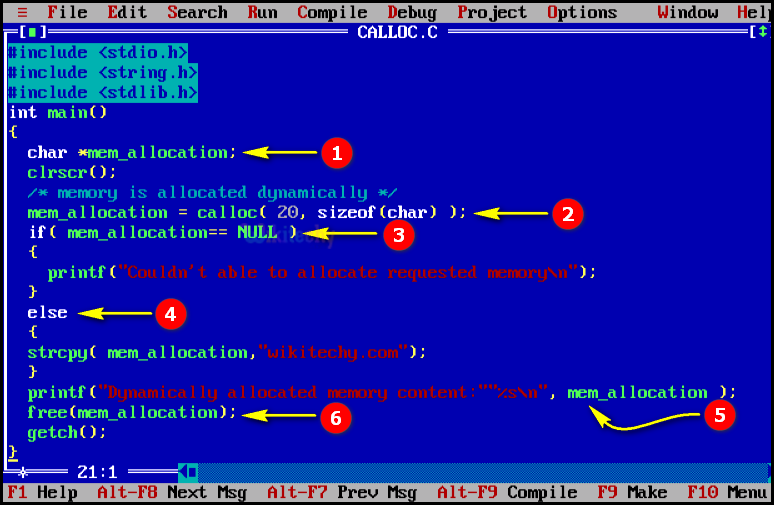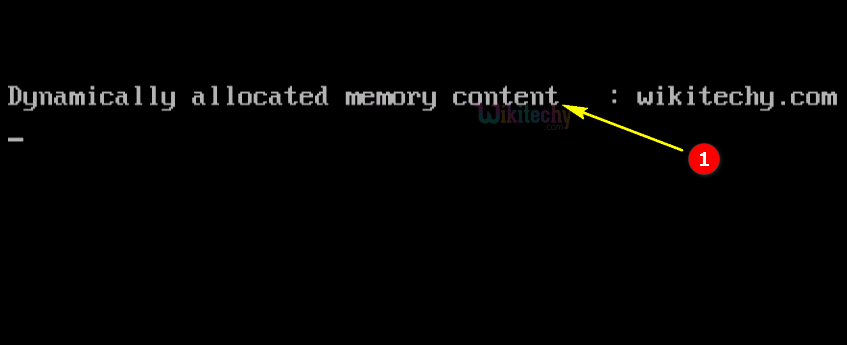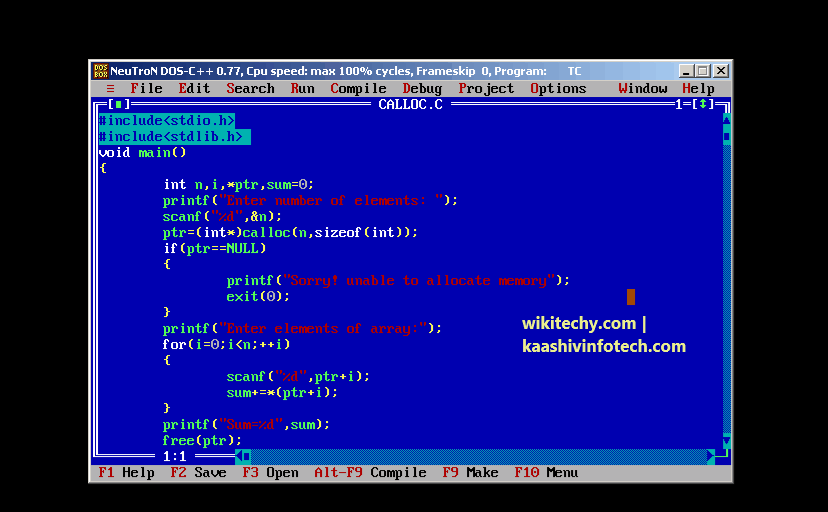# C - calloc

## Structure and Linked List with C Programming in TamilLearn C - C tutorial - calloc - C examples - C programs

## C - calloc() Function - Definition and Usage

• Calloc () function is similar to malloc function.
• But in Calloc function the memory space will be initialized before the memory allocation is set as zero.## C Syntax## C Code - Explanation1. In this statement we declare the pointer variable *ptr as integer and we assign the value for the variable “q=20” .
2. In this statement we assign the address of the “q” variable to “ptr” .
3. In this printf statement we print the address of the variable “q” .
4. In this printf statement we print the value of the variable “q” .

## Sample Output - Programming Examples1. Here in this output the dynamic memory will be allocated and the string “wikitechy.com” will be copied and printed as shown in the console window.

## Sample Code